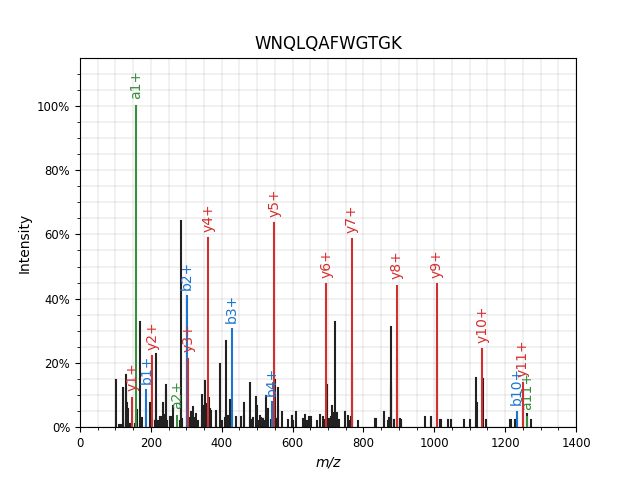# Example 4: Spectrum Annotation¶

In this example we will retrieve a spectrum and visualize it, a lot like in example 2. However, we will not read the spectrum from a file, but retrieve it directly from an online repository. We will also use Pyteomics to annotate fragment ions. Let’s get to it! Full code can be downloaded `here`.

We are going to need spectrum_utils, a library for spectrum processing and visualization. Pyteomics integrates with it and allows to create annotated spectrum plots easily.

First, we use a spectrum’s Universal Spectrum Identifier to download it directly from the MassIVE repository. Pyteomics provides tools for that in the `pyteomics.usi` module.

```from pyteomics import pylab_aux as pa, usi
import matplotlib.pyplot as plt
spectrum = usi.proxi(
'mzspec:PXD004732:01650b_BC2-TUM_first_pool_53_01_01-3xHCD-1h-R2:scan:41840',
'massive')
peptide = 'WNQLQAFWGTGK'
```

Next, just plot the spectrum and annotate it with a single function call:

```pa.annotate_spectrum(spectrum, peptide, precursor_charge=2, backend='spectrum_utils',
ion_types='aby', title=peptide)
plt.show()
```

You will see a simple spectrum_utils annotation of the spectrum:`pyteomics.pylab_aux.annotate_spectrum()` accepts a number of keyword parameters, allowing you to do just about everything you can do with `spectrum_utils`.

Note

You can omit backend=’spectrum_utils’, and `annotate_spectrum()` will use the default backend. The default backend supports less spectrum preprocessing features.

Another thing spectrum_utils lets you do is mirror plots. Following the spectrum_utils documentation, we are going to make this part more complex, showing more of what you can do. This example includes modified peptides and a bit of spectrum preprocessing. Also, this time we are going to use the spectrum_utils.iplot backend to produce an interactive plot.

To annotate the spectrum of a modified peptide, you can use the ProForma notation, which is the only format supported by spectrum_utils now. You can also use the modX notation which is standard for Pyteomics: it will be converted to ProForma if possible:

```from pyteomics import mass
peptide = 'DLTDYLoxMK'  # oxidized methionine
aa_mass = mass.std_aa_mass.copy()
aa_mass['ox'] = 15.9949  # define the mass of the label
```

Note

In this case, using aa_mass and the modX sequence, Pyteomics will convert it to a ProForma string using `pyteomics.parser.to_proforma()`:

```>>> from pyteomics import parser
>>> parser.to_proforma(peptide, aa_mass=aa_mass)
'DLTDYLM[+15.9949]K'
```

Going back to our script! Next, we choose two spectra:

```usi_top = 'mzspec:MSV000079960:DY_HS_Exp7-Ad1:scan:30372'
usi_bottom = 'mzspec:MSV000080679:j11962_C1orf144:scan:10671'

spectrum_top = usi.proxi(usi_top, 'massive')
spectrum_bottom = usi.proxi(usi_bottom, 'massive')
```

And finally, produce the image with:

```pa.mirror(spectrum_top, spectrum_bottom, peptide=peptide, precursor_charge=2,
aa_mass=aa_mass, ion_types='aby', ftol=0.5, scaling='root',
remove_precursor_peak=True, backend='spectrum_utils.iplot'
).properties(width=640, height=400).save('mirror.html')
```

Here’s the result: